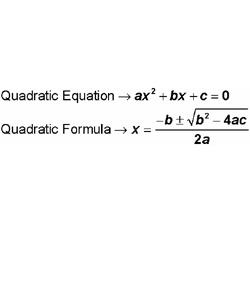3 Questions | Total Attempts: 286SettingsSolving Quadratic EquationsVOISE Algebra Unit 13 Ms. Johnson

Related Topics
• 1.
Solve. 8x2 + 10x + 3 = 0
• A.

X = -8 and -4

• B.

X = -2 and -6

• C.

X = -1.5 and -0.167

• D.

X = -0.75 and -0.5

• 2.
Solve. 1x2 + 12x + 32 = 0
• A.

X = -8 and -4

• B.

X = -2 and -6

• C.

X = -1.5 and -0.167

• D.

X = -0.75 and -0.5

• 3.
Solve. 12x2 + 20x + 3 = 0
• A.

X = -8 and -4

• B.

X = -2 and -6

• C.

X = -1.5 and -0.167

• D.

X = -0.75 and -0.5